# Composite Module (desicos.composite)¶

The desicos.composite module includes functions used to calculate laminate properties based on input data of stacking sequence and lamina properties.

The most convenient usage is probably using the desicos.composite.laminate.read_stack() function:

from desicos.composite.laminate import read_stack

laminaprop = (E11, E22, nu12, G12, G13, G23)
plyt = ply_thickness
stack = [0, 90, +45, -45]


Where the laminate stiffness matrix, the often called ABD matrix, with shape=(6, 6), can be accessed using:

>>> lam.ABD


and when shear stiffnesses are required, the ABDE matrix, with shape=(8, 8):

>>> lam.ABDE


## Composite Laminate Module (desicos.composite.laminate)¶

class desicos.composite.laminate.Laminate[source]

attribute

description

plies

list of plies

t

total thickness of the laminate

e1

equivalent laminate modulus in 1 direction

e2

equivalent laminate modulus in 2 direction

g12

equivalent laminate shear modulus in 12 direction

nu12

equivalent laminate Poisson ratio in 12 direction

nu21

equivalent laminate Poisson ratio in 21 direction

xiA

laminate parameters for extensional matrix A

xiB

laminate parameters for extension-bending matrix B

xiD

laminate parameters for bending matrix D

A

laminate extension matrix

B

laminate extension-bending matrix

D

laminate bending matrix

E

laminate transferse shear matrix

ABD

laminate ABD matrix

ABDE

laminate ABD matrix with transverse shear terms

Methods

 Use the ABDE matrix based on lamination parameters. Calculates the laminate constitutive matrix Calculates the equivalent laminate properties. Calculate the lamination parameters. Force balanced lamination parameters Force an orthotropic laminate Force a symmetric laminate Force symmetric lamination parameters
 rebuild
calc_ABDE_from_lamination_parameters()[source]

Use the ABDE matrix based on lamination parameters.

Given the lamination parameters xiA, xiB, xiC and xiD, the ABD matrix is calculated.

calc_constitutive_matrix()[source]

Calculates the laminate constitutive matrix

This is the commonly called ABD matrix with shape=(6, 6) when the classical laminated plate theory is used, or the ABDE matrix when the first-order shear deformation theory is used, containing the transverse shear terms.

calc_equivalent_modulus()[source]

Calculates the equivalent laminate properties.

The following attributes are calculated:

e1, e2, g12, nu12, nu21

calc_lamination_parameters()[source]

Calculate the lamination parameters.

The following attributes are calculated:

xiA, xiB, xiD, xiE

force_balanced_LP()[source]

Force balanced lamination parameters

The lamination parameters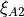and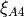are set to null to force a balanced laminate.

force_orthotropic()[source]

Force an orthotropic laminate

The terms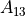,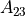,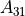,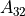,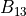,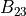,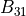,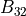,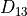,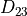,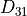,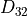are set to zero to force an orthotropic laminate.

force_symmetric()[source]

Force a symmetric laminate

The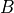terms of the constitutive matrix are set to zero.

force_symmetric_LP()[source]

Force symmetric lamination parameters

The lamination parameters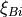are set to null to force a symmetric laminate.

desicos.composite.laminate.read_lamination_parameters(thickness, laminaprop, xiA1, xiA2, xiA3, xiA4, xiB1, xiB2, xiB3, xiB4, xiD1, xiD2, xiD3, xiD4, xiE1, xiE2, xiE3, xiE4)[source]

Calculates a laminate based on the lamination parameters.

The lamination parameters: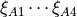,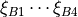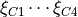,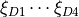are used to calculate the laminate.

Parameters
thicknessfloat

The total thickness of the laminate

laminaproptuple

The laminaprop tuple used to define the laminate material.

xiA1 to xiD4float

The 16 lamination parameters used to define the laminate.

Returns
lamLaminate

laminate with the ABD and ABDE matrices already calculated

desicos.composite.laminate.read_stack(stack, plyt=None, laminaprop=None, plyts=[], laminaprops=[])[source]

Read a laminate stacking sequence data.

An Laminate object is returned based on the inputs given.

Parameters
stacklist

Angles of the stacking sequence in degrees.

plytfloat, optional

When all plies have the same thickness, plyt can be supplied.

laminaproptuple, optional

When all plies have the same material properties, laminaprop can be supplied.

plytslist, optional

A list of floats with the thickness of each ply.

laminapropslist, optional

A list of tuples with a laminaprop for each ply.

Notes

plyt or plyts must be supplied laminaprop or laminaprops must be supplied

For orthotropic plies, the laminaprop should be:

laminaprop = (E11, E22, nu12, G12, G13, G23)


For isotropic pliey, the laminaprop should be:

laminaprop = (E, E, nu)


## Composite Lamina Module (desicos.composite.lamina)¶

class desicos.composite.lamina.Lamina[source]

attribute

description

plyid

id of the composite lamina

matobj

a pointer to a MatLamina object

t

ply thickness

theta

ply angle in degrees

L

transformation matrix for displacements to laminate csys

R

transformation matrix for stresses to laminate csys

T

transformation matrix for stresses to lamina csys

QL

constitutive matrix for plane-stress in laminate csys

laminates

laminates that contain this lamina

Methods

 rebuild

## Composite Matlamina Module (desicos.composite.matlamina)¶

class desicos.composite.matlamina.MatLamina[source]

Orthotropic material lamina

attributes

description

e1

Young Modulus in direction 1

e2

Young Modulus in direction 2

g12

in-plane shear modulus

g13

transverse shear modulus for plane 1-Z

g23

transverse shear modulus for plane 2-Z

nu12

Poisson’s ratio 12

nu13

Poisson’s ratio 13

nu23

Poisson’s ratio 23

nu21

Poisson’s ratio 21: use formula nu12/e1 = nu21/e2

nu31

Poisson’s ratio 31: use formula nu31/e3 = nu13/e1

nu32

Poisson’s ratio 32: use formula nu23/e2 = nu32/e3

rho

especific mass (mass / volume)

a1

thermal expansion coeffiecient in direction 1

a2

thermal expansion coeffiecient in direction 2

a3

thermal expansion coeffiecient in direction 3

tref

reference temperature

st1,st2

allowable tensile stresses for directions 1 and 2

sc1,sc2

allowable compressive stresses for directions 1 and 2

ss12

allowable in-plane stress for shear

strn

allowable strain for direction 1

q11

lamina constitutive constant 11

q12

lamina constitutive constant 12

q13

lamina constitutive constant 13

q21

lamina constitutive constant 21

q22

lamina constitutive constant 22

q23

lamina constitutive constant 23

q31

lamina constitutive constant 31

q32

lamina constitutive constant 32

q33

lamina constitutive constant 33

q44

lamina constitutive constant 44

q55

lamina constitutive constant 55

q66

lamina constitutive constant 66

u

matrix with lamina invariants

c

matrix with lamina stiffness coefficients

Notes

For isotropic materials when the user defines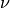and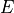,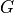will be recaculated based on equation: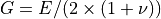; in a lower priority if the user definesand,will be recaculated based on equation: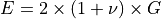.

Methods

desicos.composite.matlamina.read_laminaprop(laminaprop=None)[source]

Returns a MatLamina object based on an input laminaprop tuple.

Parameters
laminaproplist or tuple

Tuple containing the folliwing entries:

(e1, e2, nu12, g12, g13, g23, e3, nu13, nu23)

for othotropic materials the user can only supply:

(e1, e2, nu12, g12, g13, g23)

for isotropic materials the user can only supply:

(e1, e2, nu12)

symbol

value

e1

Young Module in direction 1

e2

Young Module in direction 2

nu12

12 Poisson’s ratio

g12

12 Shear Modulus

g13

13 Shear Modulus

g23

13 Shear Modulus

e3

Young Module in direction 3

nu13

13 Poisson’s ratio

nu23

23 Poisson’s ratio

Returns
matlamMatLamina

A MatLamina object.